Courses

# Carnot Heat Engine Cycle and the 2nd Law Civil Engineering (CE) Notes | EduRev

## Civil Engineering (CE) : Carnot Heat Engine Cycle and the 2nd Law Civil Engineering (CE) Notes | EduRev

The document Carnot Heat Engine Cycle and the 2nd Law Civil Engineering (CE) Notes | EduRev is a part of the Civil Engineering (CE) Course Thermodynamics.
All you need of Civil Engineering (CE) at this link: Civil Engineering (CE)

Carnot Heat Engine Cycle and the 2nd Law

In theory we may say that a heat engine absorbs a quantity of heat from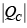high temperature reservoir at TH and rejects QC amount of heat to a colder reservoir at TH . It follows that the net work W delivered by the engine is given by: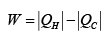.....(4.1)

Hence the efficiency of the engine is: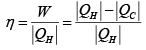.....(4.2)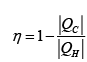.....(4.3)

Of the various forms of heat engines ideated, the Carnot engine proposed in 1824 by the French engineer Nicholas Leonard Sadi Carnot (1796-1832), provides a fundamental reference concept in the development of the second law. The so-called Carnot cycle (depicted in fig. 4.4) is a series of reversible steps executed as follows:

Step 1: A system at the temperature of a cold reservoir TC undergoes a reversible adiabatic compression which raises it temperature to that of a hot reservoir at T

Step 2: While in contact with the hot reservoir the system absorbs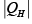amount of heat through an isothermal process during which its temperature remains at T

Step 3: The system next undergoes a reversible adiabatic process in a direction reverse of step 1 during which its temperature drops back to TC
Step 4: A reversible isothermal process of expansion at Ttransfersamount of heat to the cold reservoir and the system state returns to that at the commencement of step 1.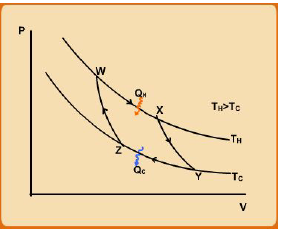Fig. 4.4 Carnot Cycle Processes

The Carnot engine, therefore, operates between two heat reservoirs in such a way that all heat exchanges with heat reservoirs occur under isothermal conditions for the system and at the temperatures corresponding to those of the reservoirs. This implies that the heat transfer occurs under infinitesimal temperature gradients across the system boundary, and hence these processes are reversible (see last paragraph of section 1.9). If in addition the isothermal and adiabatic processes are also carried out under mechanically reversible (quasi-static) conditions the cycle operates in a fully reversible manner. It follows that any other heat engine operating on a different cycle (between two heat reservoirs) must necessarily transfer heat across finite temperature differences and therefore cannot be thermally reversible. As we have argued in section that irreversibility also derives from the existence of dissipative forces in nature, which essentially leads to waste of useful energy in the conversion of work to heat. It follows therefore the Carnot cycle (which also comprises mechanically reversible processes) offers the maximum efficiency possible as defined by eqn. 4.3. This conclusion may also be proved more formally .

We next derive an expression of Carnot cycle efficiency in terms of macroscopic state properties. Consider that for the Carnot cycle shown in fig. 4.4 the process fluid in the engine is an ideal gas. Applying the eqn. 3.13 the heat interactions during the isothermal process may be shown to be: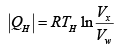...(4.4)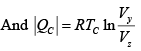...(4.5)

Further for the adiabatic paths xy and zw using eqn. 3.17 one may easily derive the following equality: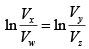...(4.6)

Using eqns. 4.4 – 4.5 we may write: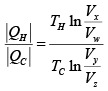...(4.7)

Finally applying eqn. (4.6) on obtains: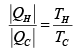...(4.8)

Hence, using eqn. 4.3: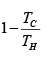...(4.9)

Also,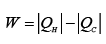...(4.10)

Eqn. (4.8) may be recast as: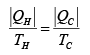...(4.10)

As heat QH enters the system it is positive, while Qleaves the system, which makes it negative in value. Thus, removing the modulus use, eqn. 4.10 may be written as: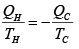...(4.11)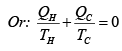...(4.12)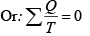...(4.13)

onsider now eqn. 4.9. For the Carnot efficiency to approach unity (i.e., 100%) the following conditions are needed: TH → ∞ ; or Tc →0.
Obviously neither situation are practicable, which suggests that the efficiency must always be less than unity. In practice, the naturally occurring bodies that approximate a cold reservoir are: atmospheres, rivers, oceans, etc, for which a representative temperature Tis ~ 3000K. The hot reservoirs, on the other hand are typically furnaces for which TH ~ 6000K. Thus the Carnot efficiency is ~ 0.5. However, in practice, due to mechanical irreversibilities associated with real processes heat engine efficiencies never exceed 40%.

It is interesting to note that the final step of thermodynamic analysis of Carnot cycle (i.e., eqn. 4.13) leads to the conclusion that there exists a quantity  Q/T which add up to zero for the complete cycle. Let us explore extending the idea to any general reversible cycle (as illustrated in fig.4.5) run by any working fluid in the heat engine.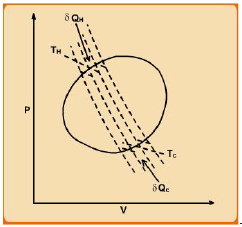ig. 4.5 Illustration of an arbitrary cycle decomposed into a series of small Carnot cycles The complete cycle may, in principle, be divided into a number of Carnot cycles (shown by dotted cycles) in series. Each such Carnot cycle would be situated between two heat reservoirs. In the limit that each cycle becomes infinitesimal in nature and so the number of such cycles infinity, the original, finite cycle is reproduced. Thus for each infinitesimal cycle the heat absorbed and released in a reversible manner by the system fluid may be written as δ |QH| and δ |QC| respectively. Thus, now invoking eqn. 4.12 for each cycle: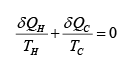...(4.14)

Hence, applying eqn. 4.13 to sum up the effects of series of all the infinitesimal cycles, we arrive at the following relation for the entire original cycle: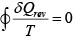...(4.15)

Clearly, the relation expressed by eqn. 4.15 suggests the existence of a state variable of the form rev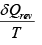as its sum over a cycle is zero. This state variable is termed as “Entropy” (S) such that: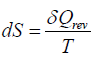...(4.16)

Thus: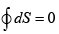Thus, for a reversible process: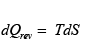...(4.17)

If applied to a perfectly reversible adiabatic process eqn. 4.16 leads to the following result: dS = 0. Thus, such a process is alternately termed as isentropic. Since entropy is a state property (just as internal energy or enthalpy), even for irreversible process occurring between two states, the change in entropy would be given by eqn. 4.16. However since entropy is calculable directly by this equation one necessarily needs to construct a reversible process by which the system may transit between the same two states. Finite changes of entropy for irreversible processes cannot be calculated by a simple integration of eqn. 4.17. However, this difficulty is circumvented by applying the concept that regardless of the nature of the process, the entropy change is identical if the initial and final states are the same for each type of process. This is equally true for any change of state brought about by irreversible heat transfer due to finite temperature gradients across the system and the surroundings. The same consideration holds even for mechanically irreversibly processes.  With the introduction of the definition of entropy the Carnot engine cycle may be redrawn on a temperature-entropy diagram as shown in fig. 4.6.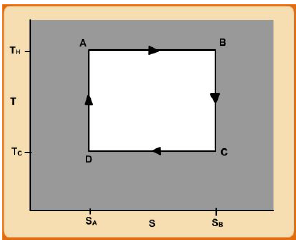Fig. 4.6 Representation of Carnot cycle on T-S diagram

Offer running on EduRev: Apply code STAYHOME200 to get INR 200 off on our premium plan EduRev Infinity!

## Thermodynamics

56 videos|92 docs|33 tests

,

,

,

,

,

,

,

,

,

,

,

,

,

,

,

,

,

,

,

,

,

;